# Solve this DE using power series b) 2(x+1)y' + y =0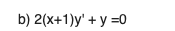Solve this DE using power series

b) 2(x+1)y' + y =0

Here I'm using power series solution answer is below any query comment and rate my answer thank you.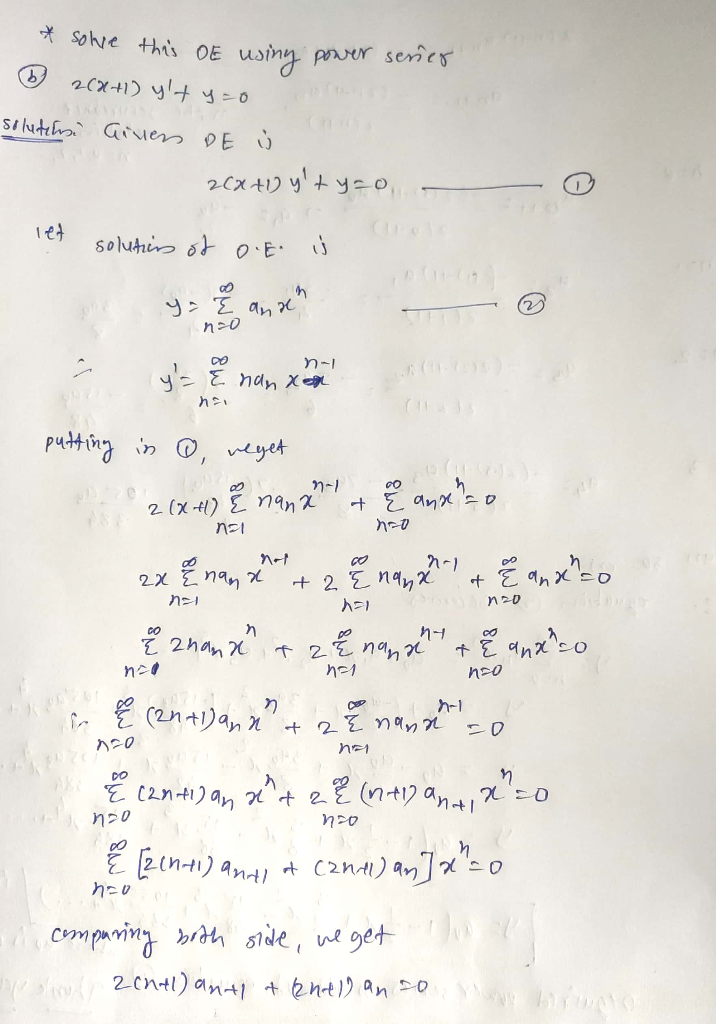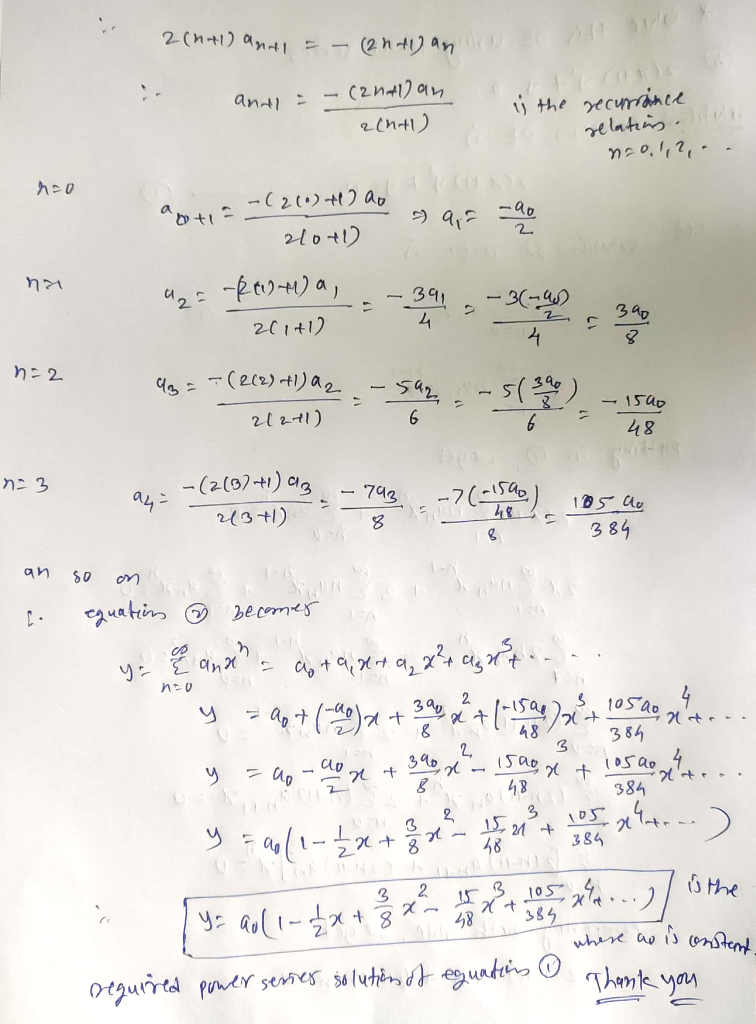##### Add Answer of: Solve this DE using power series b) 2(x+1)y' + y =0
Similar Homework Help Questions
• ### Solve y''-(x^2)y=0 using power series

I need to solve y''-(x^2)y=0 using power series and find the recursion relation.

• ### the DE 3xy', + (2-x)y'-y-0 and when r-0 С.-Cu-1. When r-1. ck-Ser. For Find two power series solutions to the D...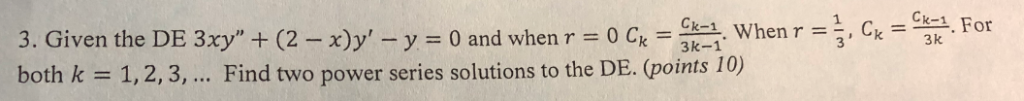the DE 3xy', + (2-x)y'-y-0 and when r-0 С.-Cu-1. When r-1. ck-Ser. For Find two power series solutions to the DE. (points 10) 3 k 1,2,3, both k the DE 3xy', + (2-x)y'-y-0 and when r-0 С.-Cu-1. When r-1. ck-Ser. For Find two power series solutions to the DE. (points 10) 3 k 1,2,3, both k

• ### 0: 1. Solve the following differential equation using a power series centered at to y" -...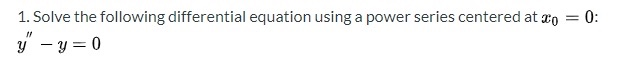0: 1. Solve the following differential equation using a power series centered at to y" - y=0

• ### use the power series to solve the differential equation (x-1)y"+y'=0

use the power series to solve the differetial equation (x-1)y"+y'=0

• ### power series of a given DE

let Y" + 4XY' -2Y = 0a.) Find the two power solutions of the given DE about the ordinarypt x=0 (Give at least 4 tearms in each power series)b)What is the formula for each of the power series solutionsc) find the solution of the IVP given by the initial conditionsY(0)=-3, Y'(0)=2I forgot everything with power series.how would I set theup?

• ### Use power series y' = 1/(2 - x) y(0) = 2

Use power series y' = 1/(2 - x) y(0) = 2

• ### Solve the initial value problems by a power series (x-2)y’=xy, y(0)=4

solve the initial value problems by a power series (x-2)y’=xy, y(0)=4

• ### n=0 4. Using the power series cos(x) = { (-1)",2 (-0<x<0), to find a power (2n)!...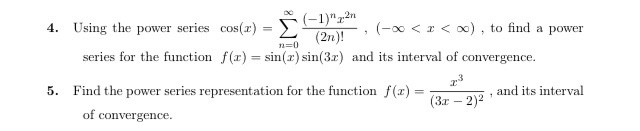n=0 4. Using the power series cos(x) = { (-1)",2 (-0<x<0), to find a power (2n)! series for the function f(x) = sin(x) sin(3x) and its interval of convergence. 23 Find the power series representation for the function f(2) and its interval (3x - 2) of convergence. 5. +

• ### 4. (a) Solve the differential equation (1 − x 2 )y ′′ − 2xy′ + λ(λ + 1)y = 0 using power series centered at 0 , in which...

4. (a) Solve the differential equation (1 − x 2 )y ′′ − 2xy′ + λ(λ + 1)y = 0 using power series centered at 0 , in which λ is a constant. Write your solution as a linear combination of two independent solutions whose coefficients are expressed in terms of λ . Compute the coefficients of each solution up to and including the x 5 term. Without computing them, what is the smallest possible value of the radius of...

• ### 4. (a) Solve the differential equation (1 − x 2 )y ′′ − 2xy′ + λ(λ + 1)y = 0 using power series centered at 0 , in which...

4. (a) Solve the differential equation (1 − x 2 )y ′′ − 2xy′ + λ(λ + 1)y = 0 using power series centered at 0 , in which λ is a constant. Write your solution as a linear combination of two independent solutions whose coefficients are expressed in terms of λ . Compute the coefficients of each solution up to and including the x 5 term. Without computing them, what is the smallest possible value of the radius of...

Free Homework App